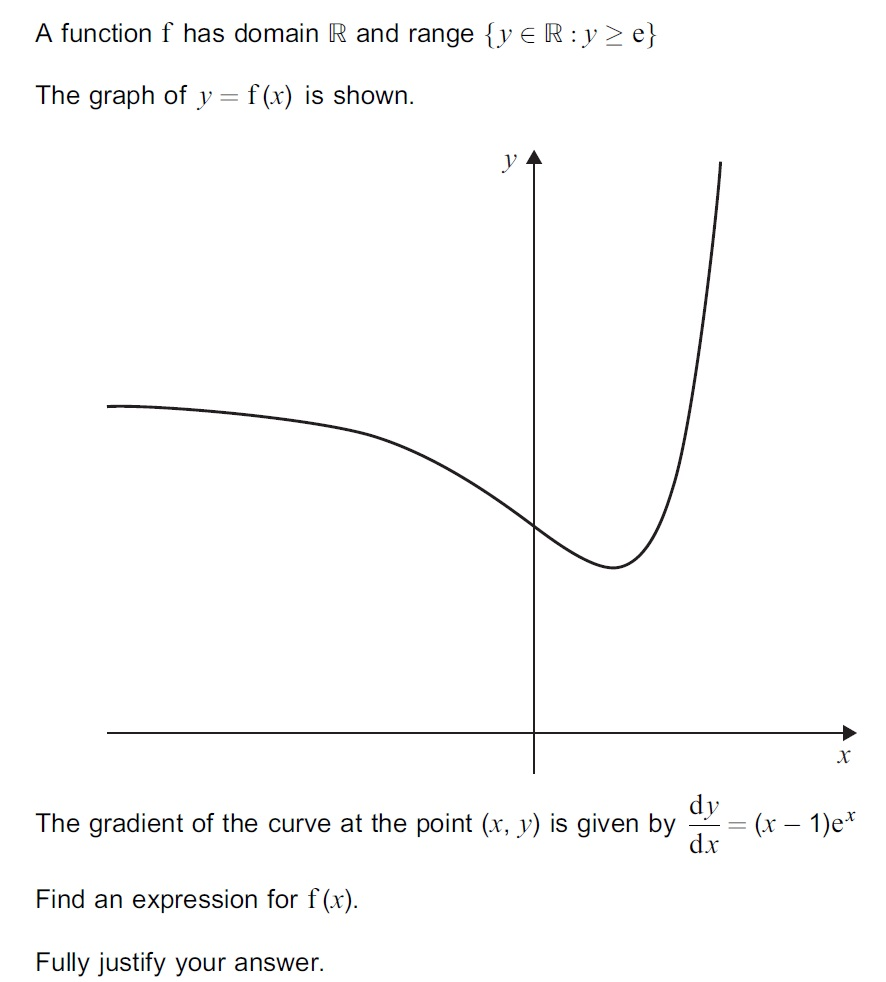# Practice Questions

1.2. A curve has equation   $y=2{x}^{2}–8x\sqrt{x}+8x+1$   for  $x\ge 0$

(a) Prove that the curve has a maximum point at (1, 3). Fully justify your answer.

(b) Find the coordinates of the other stationary point of the curve and state its nature.

3.